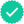College Algebra
7th Edition
ISBN: 9781305115545
Author: James Stewart, Lothar Redlin, Saleem Watson
Publisher: Cengage Learning#### Related questions#### Concept explainers

Question

How do I calculate a loan? Given the cost, the APR and how many years till its paid off (15 year loan). How much will you borrow? What is the monthly payment?

Expert SolutionStep 1

here given details are the cost, Annual percentage rate (APR) and the total tenure of the loan repayment (15 years).

We need to calculate the amount can be borrowed and monthly payment.

First step is to see the cost which we have to pay. See if you have any savings to pay some part of it and the balance amount should be taken as loan to pay the total cost.

Let us assume that the

Step 2

Once we know the amount which we are borrowing then we can calculate the monthly payment with the given information.

Let us assume the amount borrowed = L

Annual percentage rate = i%

n = total number of payments and M be the monthly paid amount.

Step 3

Here given interest rate as i% which is annual interest rate. First divide the interest rate i by 100 and write it in decimal form and then divide it by the total number of payments done in year.

Here payments are done monthly so the number of payments per year = 12

So the effective interest rate " j"= i/12 (remember i should be in decimal form)

Here the total tenure of the loan to be repaid is given as 15 years and we pay monthly, there are 12 months in a year. SO the total number of payments = 12*15 = 180 = nStep by stepSolved in 6 steps with 3 imagesKnowledge Booster••